# Quiz 16: Revenue Management With Capacity Controls

Business

Revenue management is concerned with maximizing the revenue by making optimal choice of capacity and pricing strategy given the supply or demand is fairly constant. In case of protection level/ booking limit is a capacity control tool. In this case, generally, two types of reservations are used to cater two types of customer arrivals. The arrival occurs in a staggered manner i.e. the low fare or discounted price offers are given the first priority is arrival. The concept from a newsvendor model from single period inventory model is borrowed in computing the optimal choice of capacity reserved for the full-priced offers. a. The available total capacity is 150 rooms. Since 50 rooms are reserved for full-price rooms, the available capacity for the discounted rooms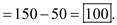b. Compute the optimal reserved capacity for the full-price rooms as follows: Full price,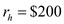Half price,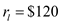For optimal reserved capacity of high-fare rooms, the probability of high-fare demand being less than or equal to reserved quantity equals the critical ratio. In other words,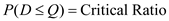Or,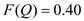Compute the value of the standard normal variable z using the following Excel function: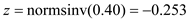With the given full-price demand distribution, compute the value of Q using the following formula:Rounding off the value of Q , the optimal level of full-priced rooms is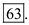c. After responding to the competitor's move the prices are as following: Full price,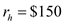Half price,Since the critical ratio reduces, the protection level for the full-price will also reduce. d. Given the protection level, compute the left-over inventory level as follows: Protection level, Q = 61The corresponding loss function,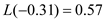Expected lost sales,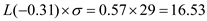So,The expected left-over inventory is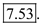e. Note that on an average, 70 full-price rooms will be booked. Compute the expected revenue using the following equation:So, the expected revenue will be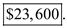f. If there is no protection for full-price rooms, then all rooms will be sold as discounted-priced. So, the expected revenue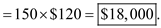g. Given the protection level, compute the expected revenue as follows: Protection level, Q = 50The corresponding loss function from the loss function table,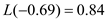Expected lost sales,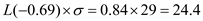So,So, the expected revenue is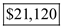.

Revenue management is concerned with maximizing the revenue by making optimal choice of capacity and pricing strategy given the supply or demand is fairly constant. In case of overbooking strategy, the overbooking is done for regular-price reservations. Overbooking is deployed only when the customer reservation is not deterministic in nature and as a result, a portion of the customer do not show up for their reserved seats. a. Compute the optimum overbooking limit using the following formula:Where,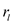is the price of the regular-fare rooms. For optimal overbooking limit, the probability of demand being less than or equal to reserved quantity equals the critical ratio. In other words,Or,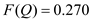From the given Poisson table, F (13) = 0.3171 0.270 So, the optimal overbooking limit is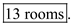b. The booked number of rooms is 160. So, if 9 or more no-shows happens, the hotel will not be able to completely accommodate the demand. From the Poisson table, F (9) = 0.0552. So, there is a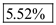chance that the demand cannot be fulfilled. c. The booked number of rooms is 165. So, if 15 or fewer no-shows happen, the hotel will be fully occupied and not able to completely accommodate the demand. From the Poisson table, F (15) = 0.5170. So, the probability that the Inn is fully occupied is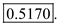d. The overbooking limit is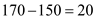. Corresponding to this value, the loss function is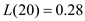So,So, the expected cost of bumping =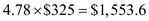Therefore, the expected cost of bumping is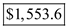.

Revenue management is concerned with maximizing the revenue by making optimal choice of capacity and pricing strategy given the supply or demand is fairly constant. In case of protection level/ booking limit is a capacity control tool. In this case, generally, two types of reservations are used to cater two types of customer arrivals. The arrival occurs in a staggered manner i.e. the low fare or discounted price offers are given the first priority is arrival. The concept from a newsvendor model from single period inventory model is borrowed in computing the optimal choice of capacity reserved for the full-priced offers. a. Compute the optimal quantity sold in advance as follows: High price,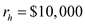Regular price,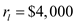For optimal reserved capacity of high price, the probability of high-price demand being less than or equal to reserved quantity equals the critical ratio. In other words,Or,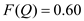Compute the cumulative probability from the given discrete distribution of number of slots using the Excel formulation as follows: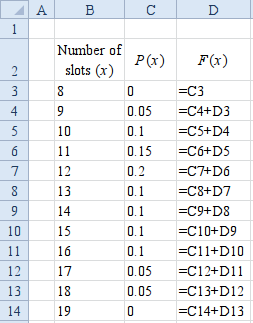Result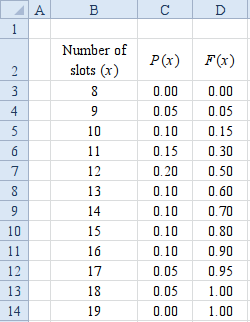With the given high-price demand cumulative distribution as shown above, F(13) = 0.60 So, the optimal level of high-priced booking is 13. So, the optimal quantity that is to be sold in advance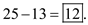b. Compute the optimal quantity sold in advance after altering the critical ratio based on the given salvage value of the slots. High price,Regular price,For optimal reserved capacity of high price, the probability of high-price demand being less than or equal to reserved quantity equals the critical ratio. In other words,Or,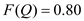With the given high-price demand cumulative distribution, F(15) = 0.60 So, the optimal level of high-priced booking is 15. So, the optimal quantity that is to be sold in advance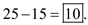c. Given the number of slots booked in advance equal to 10, compute the probability of stock left-over for the stand-by customers as follows: So, high-price booking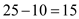. There will be stand-by customers if 14 or less slots are booked. From the cumulative distribution table, F(14) = 0.70 So, the probability of stock left-over for the stand-by customers is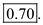d. Compute the optimum overbooking limit using the following formula: Cost of underage,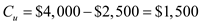Cost of underage,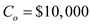For optimal overbooking limit, the probability of demand being less than or equal to reserved quantity equals the critical ratio. In other words,Or,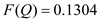From the given Poisson table for the mean of 9, F(6) = 0.2068 0.1304 So, the optimal overbooking limit is 6 slots. So, the number of slots to be booked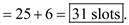e. Compute the optimum overbooking limit using the following formula: Cost of underage,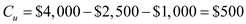Cost of underage,For optimal overbooking limit, the probability of demand being less than or equal to reserved quantity equals the critical ratio. In other words,Or,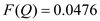From the given Poisson table for the mean of 4.5, F(6) = 0.0611 0.0476 So, the optimal overbooking limit is 1 slot only. So, the number of slots to be booked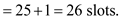Therefore, the optimal overbooking quantity is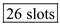.